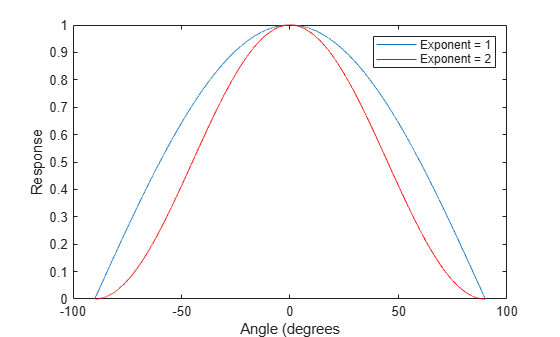Main Content

## Cosine Antenna Element

### Support for Cosine Antenna Elements

The `phased.CosineAntennaElement` object models an antenna element whose response follows a cosine function raised to a specified power in both the azimuth and elevation directions.

The object returns the field response (also called field pattern)

`$f\left(az,el\right)={\mathrm{cos}}^{m}\left(az\right){\mathrm{cos}}^{n}\left(el\right)$`

of the cosine antenna element.

In this expression

• az is the azimuth angle.

• el is the elevation angle.

• The exponents m and n are real numbers greater than or equal to zero.

The response is defined for azimuth and elevation angles between –90° and 90°, inclusive, and is always positive. There is no response at the backside of a cosine antenna. The cosine response pattern achieves a maximum value of 1 at 0° azimuth and 0° elevation. Larger exponent values narrow the response pattern of the element and increase the directivity.

The power response (or power pattern) is the squared value of the field response.

`$P\left(az,el\right)={\mathrm{cos}}^{2m}\left(az\right){\mathrm{cos}}^{2n}\left(el\right)$`

When you use the cosine antenna element, you specify the exponents of the cosine pattern using the `CosinePower` property and the operating frequency range of the antenna using the `FrequencyRange` property.

### Concentrating Cosine Antenna Response

This example shows the effect of concentrating the cosine antenna response by increasing the exponent of the cosine factor. The example computes and plots the cosine response for exponents equal to 1 and 2 for a single angle between -90 and 90 degrees. The angle can represent azimuth or elevation.

```theta = -90:.01:90; costh1 = cosd(theta); costh2 = costh1.^2; plot(theta,costh1) hold on plot(theta,costh2,'r') hold off legend('Exponent = 1','Exponent = 2','location','northeast'); xlabel('Angle (degrees') ylabel('Response')```### Plot 3-D Response of Cosine Antenna Element

This example shows how to construct an antenna with a cosine-squared response in both azimuth and elevation. The operating frequency range of the antenna is 1 to 10 GHz. Plot the 3-D antenna response at 5 GHz.

```sCos = phased.CosineAntennaElement(... 'FrequencyRange',[1 10]*1e9,'CosinePower',[2 2]); pattern(sCos,5e9,[-180:180],[-90:90],'CoordinateSystem',... 'Polar','Type','powerdb')```## Support

#### Exploring Hybrid Beamforming Architectures for 5G Systems

Download white paper